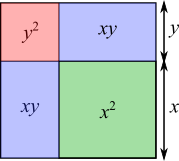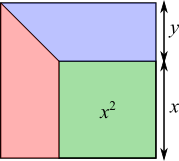#### You may also like### Golden Thoughts

Rectangle PQRS has X and Y on the edges. Triangles PQY, YRX and XSP have equal areas. Prove X and Y divide the sides of PQRS in the golden ratio.### Two Cubes

Two cubes, each with integral side lengths, have a combined volume equal to the total of the lengths of their edges. How big are the cubes? [If you find a result by 'trial and error' you'll need to prove you have found all possible solutions.]### Square Mean

Is the mean of the squares of two numbers greater than, or less than, the square of their means?

# A Third of the Area

##### Age 14 to 16 Short Challenge Level:

Answer: $\dfrac{1}{\sqrt3-1} = \dfrac{1+\sqrt3}2 =1.366$ to 3 d.p.

Using the relationship between the areas
The area of the small square is $\frac13$ of the area of the large square, so $$\frac {x^2}{(x+y)^2}=\frac13\Rightarrow\left(\frac x{x+y}\right)^2=\frac13\Rightarrow\frac x{x+y}=\dfrac1{\sqrt3}$$
We can make $\dfrac x y$ the subject of this equation:
\begin{align}\frac{x}{x+y}&=\frac1{\sqrt3}\\ \Rightarrow\sqrt3x&=x+y\\ \Rightarrow\sqrt3x-x&=y\\ \Rightarrow(\sqrt3-1)x&=y\\ \Rightarrow\frac x y &=\frac 1 {\sqrt3-1}\end{align}
To see how to express this as a fraction with a whole number denominator, see the bottom of the solution.

Splitting the larger square into smaller squares and rectanglesGreen = $\frac13$ of total
$\therefore$ red + blue = green $\times$ 2

$\Rightarrow y^2+2xy=2x^2$
$\Rightarrow 1+2\dfrac xy=2\dfrac{x^2}{y^2}$

Let $\dfrac xy=w$:
\begin{align}1+2w=2w^2&\Rightarrow 2w^2-2w-1=0\\ &\Rightarrow w^2-w-\tfrac12=0\\ &\Rightarrow\left(w-\tfrac12\right)^2-\tfrac14-\tfrac12=0\\ &\Rightarrow w-\tfrac12=\pm\tfrac{\sqrt3}{2}\\ &\Rightarrow w=\tfrac12\pm\tfrac{\sqrt3}{2}\text{ take the positive root since }w>0\\ &\Rightarrow w=\tfrac{1+\sqrt3}2\end{align}

Splitting the larger square into trapeziumsThe area of each trapezium is $\frac12\left(\left(x+y\right)+x\right)y$

Green square occupies a third of the area
$\therefore$ the three shapes have equal area

$\frac12\left(\left(x+y\right)+x\right)y=x^2$

Multiplying both sides of this equation by $2$ and expanding gives $2xy+y^2=2x^2$.

$\dfrac xy$ can be found from this equation as shown above.

You can find more short problems, arranged by curriculum topic, in our short problems collection.